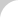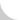The Complete Guide to Chain# 2.3.2 Dynamic Tension of Starting and Stopping

Conveyor chain accelerates when it changes from stop mode to operational speeds, and decelerates when it changes from operational speeds to stop modes. Therefore, a dynamic tension resulting from inertia affects the conveyor chain, and it is added to "the tension produced when conveyed objects are moving at fixed speed," which is discussed in Basics Section 2.3.1. You must consider dynamic tension caused by inertia, especially in the following cases:

1. Starting and stopping chains frequently, such as intermittent use with indexing equipment.
2. Starting and stopping in very short time spans.
3. When chains in motion suddenly receive stationary objects to convey.

The dynamic tension by inertia is calculated with this formula:

T1 = M × α = M × (dv / dt)

Where:

• M = total weight of conveying apparatus, including chain, attachments, product, etc., (kg)
• α = maximum acceleration (m/s2)
• dv = change in speed (m/s)
• dt = time in which speed change occurs (s)

For example:

• M = 5,000 kg, the total weight of chain, attachment, product, etc.
• f = 0.12, the dynamic coefficient of friction
• T = 5,000 × 9.8 × 0.12 = 5,880 N

This assumes the conveyor is operating at constant speed. But when the chain starts, if the speed is increased to 20 m/min. in 0.2 seconds, then:

dv = 20/60 = 0.33 m/s

dt = 0.2 s

T1 = 5,000 × (0.33 / 0.2) = 8,250 N

Maximum tension = T + T1 = 14,130 N

If the chain is accelerated frequently in this manner, then select chains using T + T1.